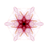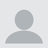首页   注册   登录
V2EX = way to explore
V2EX 是一个关于分享和探索的地方

V2EX 提问指南
V2EX  ›  问与答

# 求助 cs 的课程,先做得像这个样子, 以后还要加上一些小功能改善这个小游戏： https://youtu.be/YQG-PPxig-k 不知道何从下手，求大佬们帮帮忙,目前已有的 code:

KonoDio · 320 天前 · 524 次点击

public class Battleship extends ConsoleProgram { public void run() { System.out.println("======================"); System.out.println("Welcome to battleship."); System.out.println("======================"); } }

public class Grid { private Location[][] grid;

``````// Constants for number of rows and columns.
public static final int NUM_ROWS = 10;
public static final int NUM_COLS = 10;
// Create a new Grid. Initialize each Location in the grid
// to be a new Location object.
public Grid()
{
grid = new Location;
for(int row = 0; row < NUM_ROWS; row++)
{
for(int col = 0; col < NUM_COLS; col++)
{
grid[row][col] = new Location();
}
}
}

// Mark a hit in this location by calling the markHit method
// on the Location object.
public void markHit(int row, int col)
{
grid[row][col].markHit();
}    // Mark a miss on this location.
public void markMiss(int row, int col)
{
grid[row][col].markMiss();
}

// Set the status of this location object.
public void setStatus(int row, int col, int status)
{
grid[row][col].setStatus(status);
}

// Get the status of this location in the grid
public int getStatus(int row, int col)
{
return grid[row][col].getStatus();
}

// Return whether or not this Location has already been guessed.
public boolean alreadyGuessed(int row, int col)
{
return grid[row][col].isUnguessed() == false;
}

// Set whether or not there is a ship at this location to the val
public void setShip(int row, int col, boolean exist)
{
grid[row][col].setShip(exist);
}

// Return whether or not there is a ship here
public boolean hasShip(int row, int col)
{
return grid[row][col].hasShip();
}

// Get the Location object at this row and column position
public Location get(int row, int col)
{
return grid[row][col];
}

// Return the number of rows in the Grid
public int numRows()
{
return NUM_ROWS;
}

// Return the number of columns in the grid
public int numCols()
{
return NUM_COLS;
}
public void printStatus()
{
System.out.println("1 2 3 4 5 6 7 8 9 10");
for(int row = 0; row < NUM_ROWS; row++)
{
System.out.print((char)(row + 65));
for(int col = 0; col < NUM_COLS; col++)
{
if(grid[row][col].checkHit())
{
System.out.print(" X");
}
else if(grid[row][col].getStatus() == 2)
{
System.out.print(" O");
}
else
{
System.out.print(" -");
}
}
System.out.print(" ");
System.out.println();
}
}
public void printShips()
{
System.out.println("1 2 3 4 5 6 7 8 9 10");
for(int row = 0; row < NUM_ROWS; row++)
{
System.out.print((char)(row + 65));
for(int col = 0; col < NUM_COLS; col++)
{
if(grid[row][col].hasShip() == true)
{
System.out.print(" X");
}
else
{
System.out.print(" -");
}
}
System.out.print(" ");
System.out.println();
}
}
``````

}

public class Player { private static final int[] SHIP_LENGTHS = {2, 3, 3, 4, 5}; public Grid enemyGrid; public Grid myGrid; private static int c = 0; public Player() { myGrid = new Grid(); enemyGrid = new Grid(); } public void chooseShipLocation(Ship s, int row, int col, int direction) { if(c < 5) { s.setLocation(row, col); s.setDirection(direction); myGrid.addShip(s); c ++; } }

``````public void printMyShips()
{
myGrid.printShips();
}
public void printOpponentGuesses()
{
myGrid.printStatus();
}
public void printMyGuesses()
{
myGrid.printStatus();
}
public void recordOpponentGuess(int row,int col)
{
if(myGrid.hasShip(row, col))
{
myGrid.markHit(row, col);
}
else
{
myGrid.markMiss(row, col);
}
}
``````

}

import java.util.*;

public class Randomizer{

``````public static Random theInstance = null;

public Randomizer(){

}

public static Random getInstance(){
if(theInstance == null){
theInstance = new Random();
}
return theInstance;
}

/**
* Return a random boolean value.
* @return True or false value simulating a coin flip.
*/
public static boolean nextBoolean(){
return Randomizer.getInstance().nextBoolean();
}

/**
* This method simulates a weighted coin flip which will return
* true with the probability passed as a parameter.
*
* @param	probability	The probability that the method returns true, a value between 0 to 1 inclusive.
* @return True or false value simulating a weighted coin flip.
*/
public static boolean nextBoolean(double probability){
return Randomizer.nextDouble() < probability;
}

/**
* This method returns a random integer.
* @return A random integer.
*/
public static int nextInt(){
return Randomizer.getInstance().nextInt();
}

/**
* This method returns a random integer between 0 and n, exclusive.
* @param n	The maximum value for the range.
* @return A random integer between 0 and n, exclusive.
*/
public static int nextInt(int n){
return Randomizer.getInstance().nextInt(n);
}

/**
* Return a number between min and max, inclusive.
* @param min	The minimum integer value of the range, inclusive.
* @param max	The maximum integer value in the range, inclusive.
* @return A random integer between min and max.
*/
public static int nextInt(int min, int max){
return min + Randomizer.nextInt(max - min + 1);
}

/**
* Return a random double between 0 and 1.
* @return A random double between 0 and 1.
*/
public static double nextDouble(){
return Randomizer.getInstance().nextDouble();
}

/**
* Return a random double between min and max.
* @param min The minimum double value in the range.
* @param max The maximum double value in the rang.
* @return A random double between min and max.
*/
public static double nextDouble(double min, double max){
return min + (max - min) * Randomizer.nextDouble();
}
``````

}

public class Ship { public int length; public int direction; public int row; public int col; public static final int UNSET = -1; public static final int HORIZONTAL = 0; public static final int VERTICAL = 1; // Constructor. Create a ship and set the length. public Ship(int length) { this.row = UNSET; this.col = UNSET; this.length = length; this.direction = UNSET; }

``````// Has the location been initialized
public boolean isLocationSet()
{
return !(this.row == UNSET)&&(this.col == UNSET);
}

// Has the direction been initialized
public boolean isDirectionSet()
{
return direction != UNSET;
}

// Set the location of the ship
public void setLocation(int row, int col)
{
this.row = row;
this.col = col;
}

// Set the direction of the ship
public void setDirection(int direction)
{
this.direction = direction;
}

// Getter for the row value
public int getRow()
{
return row;
}

// Getter for the column value
public int getCol()
{
return col;
}

// Getter for the length of the ship
public int getLength()
{
return length;
}

// Getter for the direction
public int getDirection()
{
return direction;
}

// Helper method to get a string value from the direction
private String directionToString()
{
String exa = "";
if(direction == VERTICAL)
{
exa = "vertical";
}
else if(direction == HORIZONTAL)
{
exa = "horizontal";
}
else
{
exa = "unset direction";
}
return exa;
}

// Helper method to get a (row, col) string value from the location
private String locationToString()
{
String ini = "";
if(row != UNSET)
{
ini = "(" + row + ", " + col + ")";
}
else
{
ini = "(unset location)";
}
return ini;
}

// toString value for this Ship
public String toString()
{
return directionToString() + " ship of length " + length + " at "locationToString();
}
``````

}

public class Location { public boolean exist; public int status; public static final int UNGUESSED = 0; public static final int HIT = 1; public static final int MISSED = 2; // Location constructor. public Location() { this.exist = exist; this.status = status; } // Was this Location a hit? public boolean checkHit() { return status == HIT; } // Was this location a miss? public boolean checkMiss() { return status == MISSED; } // Was this location unguessed? public boolean isUnguessed() { return status == UNGUESSED; } // Mark this location a hit. public void markHit() { this.status = HIT; } // Mark this location a miss. public void markMiss() { this.status = MISSED; } // Return whether or not this location has a ship. public boolean hasShip() { return exist; } // Set the value of whether this location has a ship. public void setShip(boolean exist) { this.exist = exist; } // Set the status of this Location. public void setStatus(int status) { this.status = status; } // Get the status of this Location. public int getStatus() { return status; } }

5 回复  |  直到 2019-01-28 12:13:45 +08:001 Fanechka   319 天前 你上传 github 发个链接不就完事儿了2 bumz   319 天前3 Yourshell   319 天前 这不就扫雷么，主要就是二维数组的应用吧。4 KonoDio   317 天前 @Fanechka 去那里发什么链接啊？5 KonoDio   317 天前 @Yourshell 我没有基础